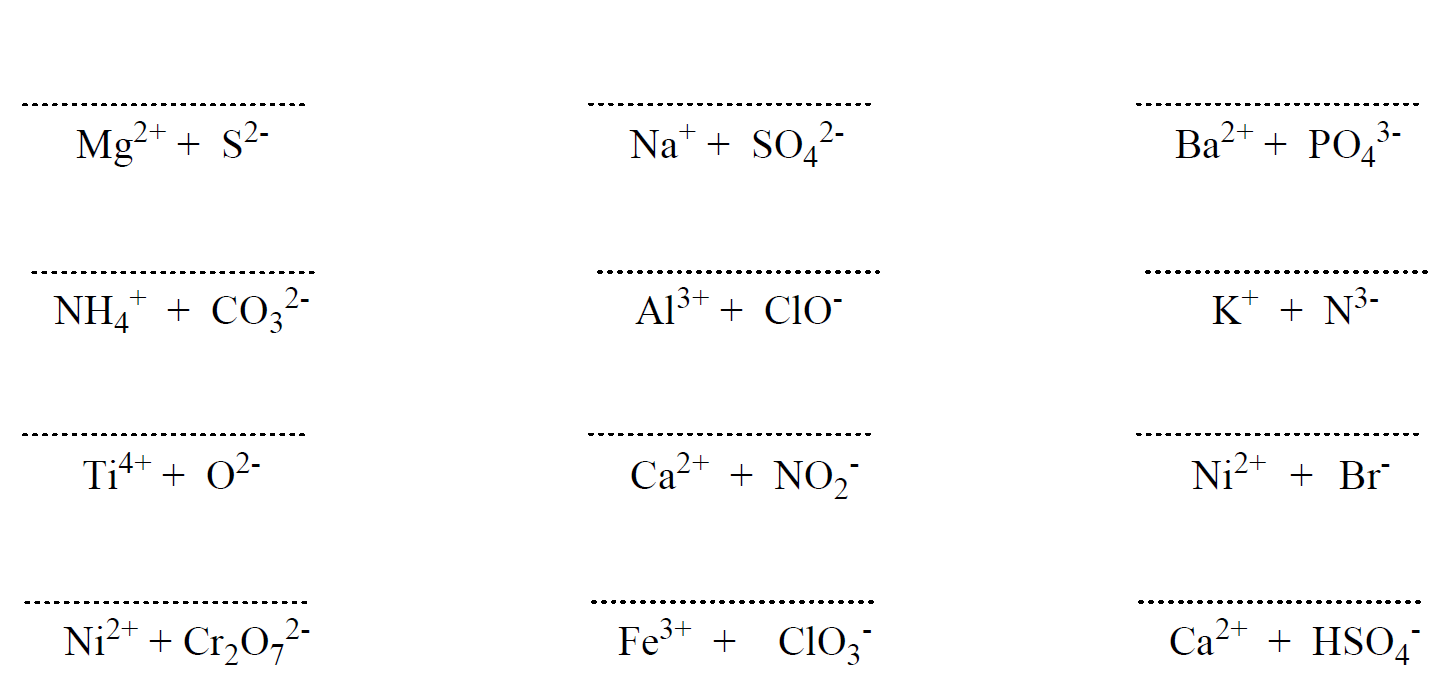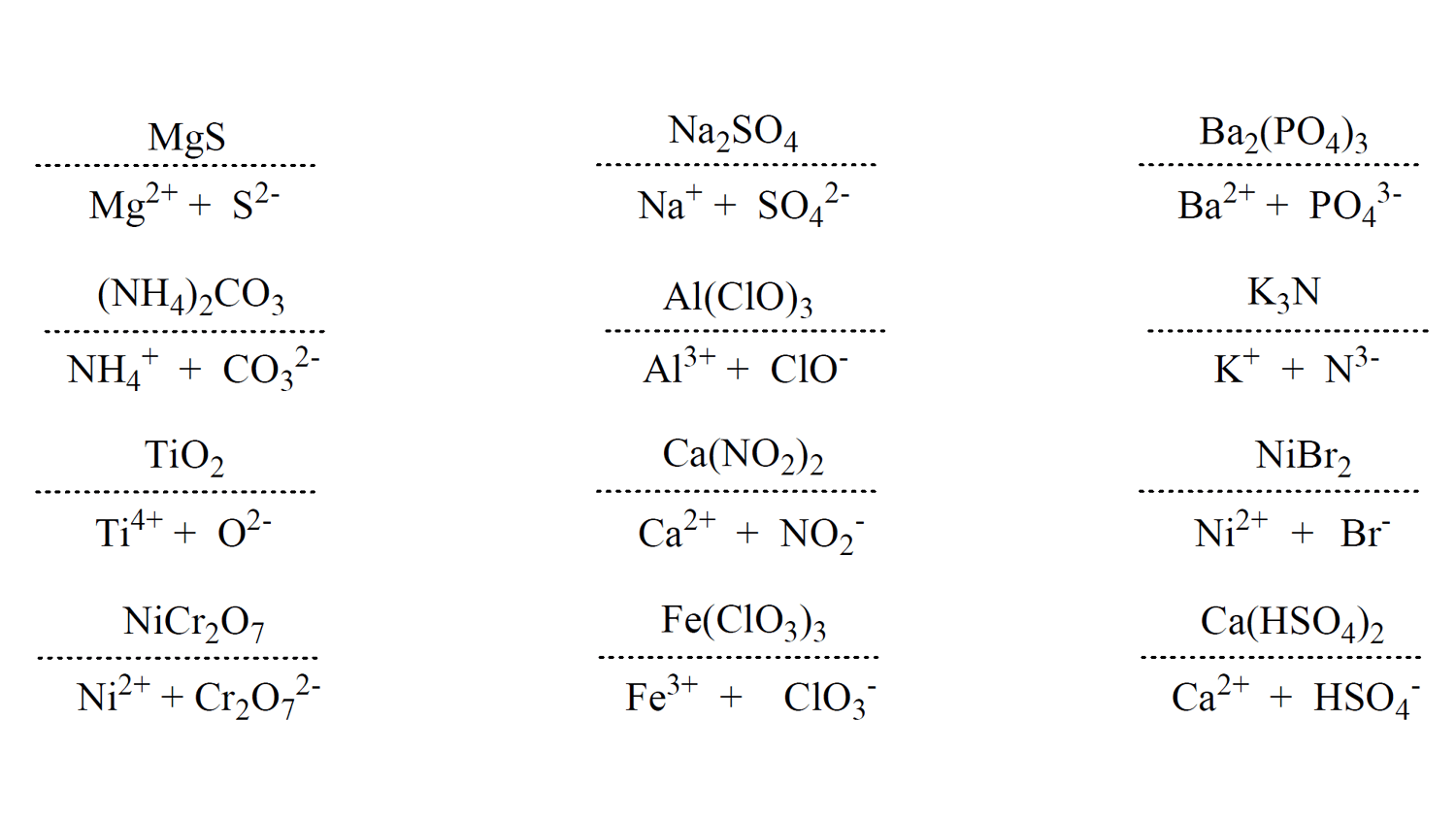# Some Patterns of Ions

To write the name of an ionic compound based on its name, we first need to remember the valency and the names of the common ions. Let’s start with the alkali and alkaline earth metals. These are on the left side of the periodic table – groups 1 and 2 and tend to form cations with charges according to their group number.

For example, all the metals in group 1 such as Na, K, Li form 1+ cations. The ones in group 2 form cations with a 2+ charge, e.g. Mg2+, Ca2+, Ba2+. Another good news about these is that their ions inherit the name of the elopement. So, the N+ ion is called sodium when naming a compound.

Aside from the alkali and alkaline earth metals, there are some transition metals and nonmetals that form ions with predictable charges. For example, halogens form anions with 1- charges and take the ending -ide:# Writing the Formulas of Compounds with Monoatomic Ions

For example, what is the formula of lithium iodide?

First, we need to come up with the correct formula of the ions. In this case, we have Li+ and I. Next, we want to determine how many of each ion we need in the formula of the salt. For this, place the ions next to each other:Remember, the idea is that the charges must be balanced making a neutral structure. So, for every +, we need a negative charge. In this example, having one of each ion will cancel the charges, and therefore, the formula would be LiI: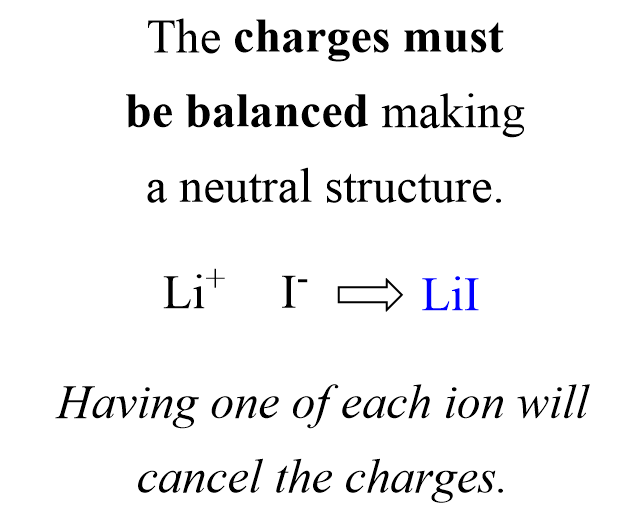Notice that we do not write subscript 1.

Let’s now write the formula for magnesium iodide. The ions are Mg2+ and I:We have 2+ and 1- charges, and therefore, we need an extra negative charge to make the structure neutral. And we achieve this by combining two iodide ions with one Mg2+: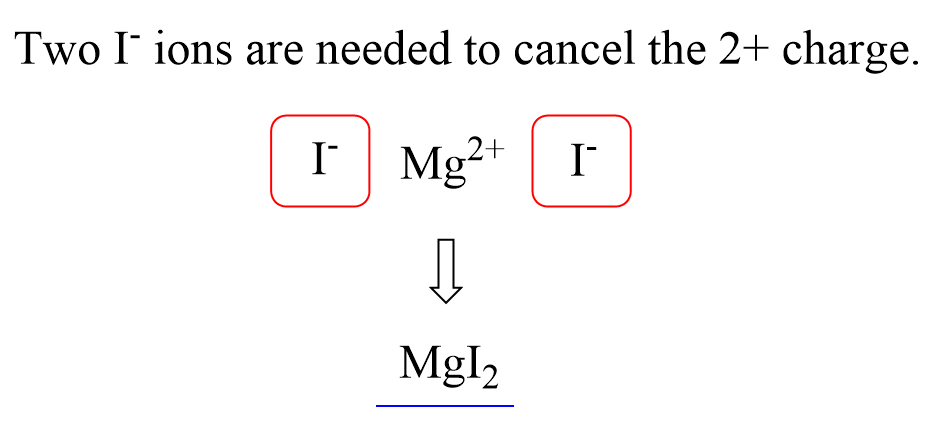Therefore, the formula will be MgI2.

# Is There is Shortcut in Writing Formulas?

Yes, there is one, and that is crossing the charge values down to the opposite ion which allows not to count the charges one by one. For this example, the 2 on top of Mg crosses to the I, and the 1 on top of the iodide crosses down to the Mg:Let’s now do an example where none of the ions has 1 charge. For example, what is the formula for aluminum sulfide?

Aluminum is in group 3, and therefore, the cation is Al3+, and the sulfide ion is the monoatomic ion of sulfur with a 2- charge: S2-. So, place these ions and cross down the charges to get the subscript for each element: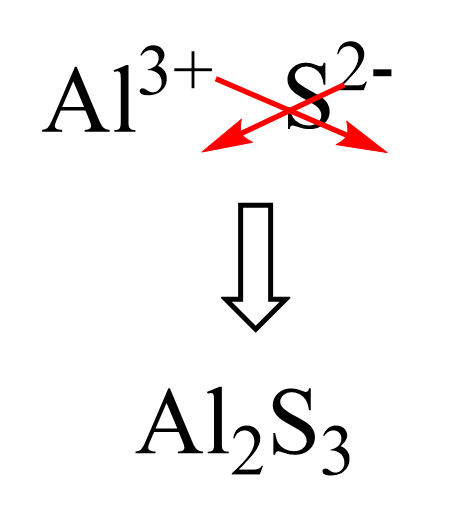The meaning of this formula is that for every 2 Al3+, we have 3 S2- ions, and that balances the overall charge to zero. Mathematically, we can write it as 2 (+3) = 3 (-2).

Below are some more examples of compounds with monoatomic ions:

K2S – Potassium sulfide, CaBr2 – Calcium bromide , AlCl3 – Aluminum chloride, MgO – Magnesium oxide

# Formulas of Compounds with Polyatomic Ions

The same principle of listing the names of the cation followed by the name of the anion applies to naming ionic compounds with polyatomic ions. The main challenge here is remembering all these ions, but you can get them with some practice.

Here is the table with the names and formulas of polyatomic ions: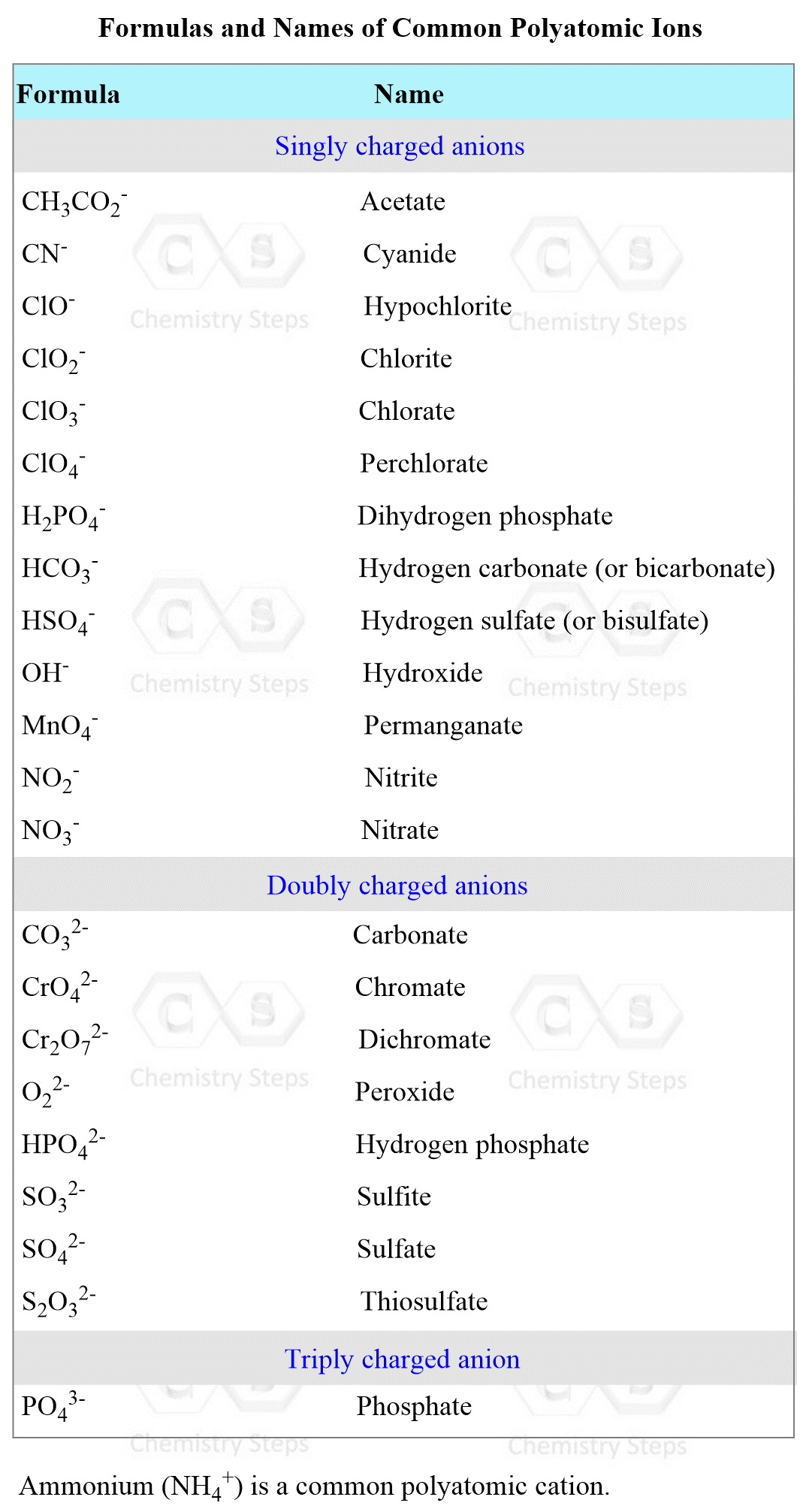For example, write the formula of barium sulfite.

Barium is in group two and therefore, the cation is Ba2+. The formula of sulfate ion is SO32- and because both ions have the same charge value, the formula of the salt would be BaSO3.

Another example: What is the formula of aluminum sulfate?

First, we know that the aluminum ion has a 3+ charge. You need to know the common polyatomic ions from where we find that sulfate is the SO42- ion. Next, we write the ions next to each other and add the correct subscripts: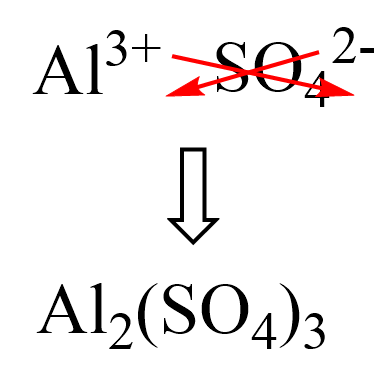Here are some more examples of compounds with polyatomic ions.

Don’t forget to add the prefix hypo– (meaning “less than”) and per– ( “more than”) for oxyanions with more than two formulas.

For example,

Ba(ClO)2 – Barium hypochlorite

Ba(ClO2)2 – Barium chlorite

Ba(ClO3)2 – Barium chlorate

Ba(ClO4)2 – Barium perchlorate

# Writing Formulas with Transition Metals

If the compound contains a cation of a transition metal with two possible charges, then a Roman numeral or the corresponding suffix is used to indicate its charge.

The suffix for the cation with a higher charge is “ic” and for the lower charge is “ous”.

The names and formulas of metals forming different cations are summarized in the table below:For example, what is the formula of ferric chloride?

The ending “ic” indicates that we have the cation with higher charge which is 3+ for iron. Crossing down the charges we get: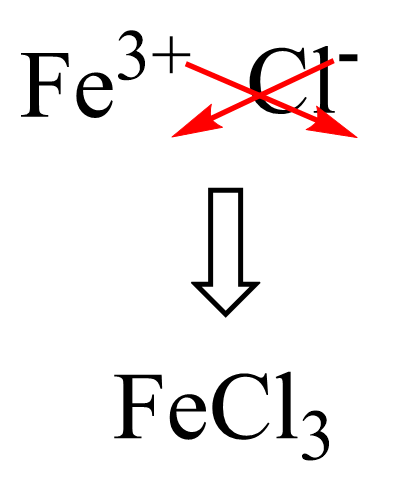The other salt with the ferrous ion would be FeCl2 – Iron(II) chloride or Ferrous chloride.

# Writing the Formulas of Hydrates

Hydrates contain a specific number of water molecules attached to them. The number of water molecules is represented with a dot and the Greek prefixes:

CuSO4 · 5H2O copper(II) sulfate pentahydrate

MgSO4 · 7H2O – magnesium sulfate heptahydrate

Notice that the number of water molecules is given by a prefix.

For example, write the formula of calcium chloride hexahydrate.

First, the formula of the salt itself: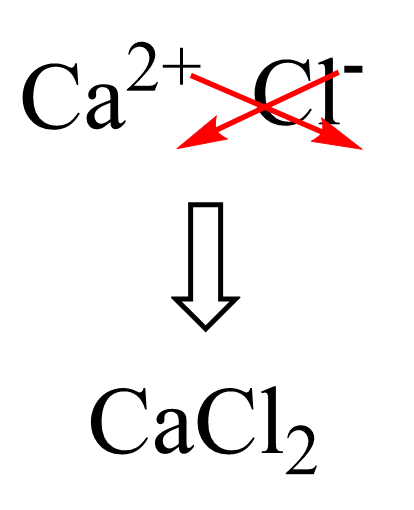Next, we add 6 water molecules according to the prefix “hexa”: CaCl2 · 6H2O

Check Also

#### Practice

1.

Write the formula for each compound: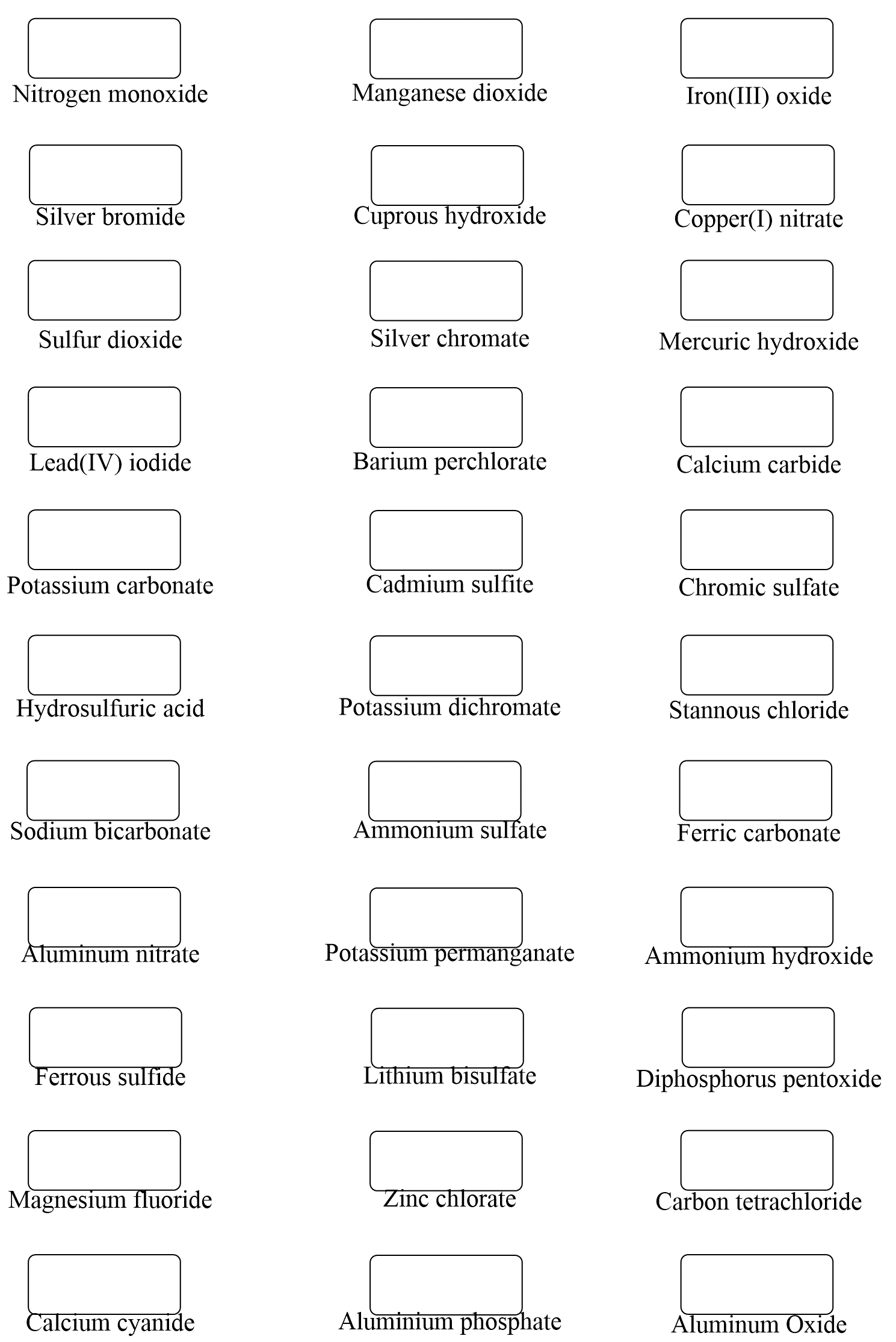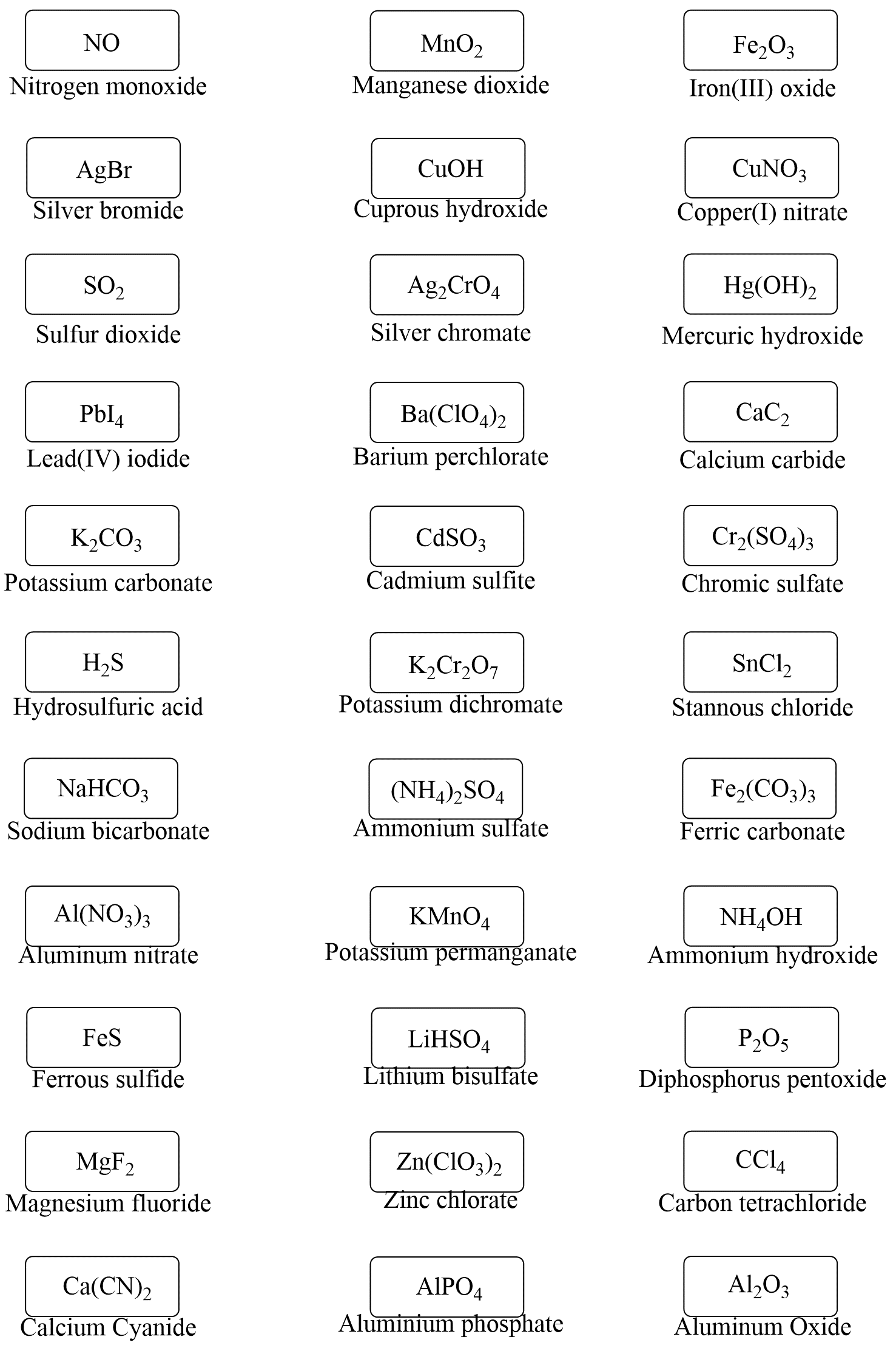2.

Write an acceptable name for each compound: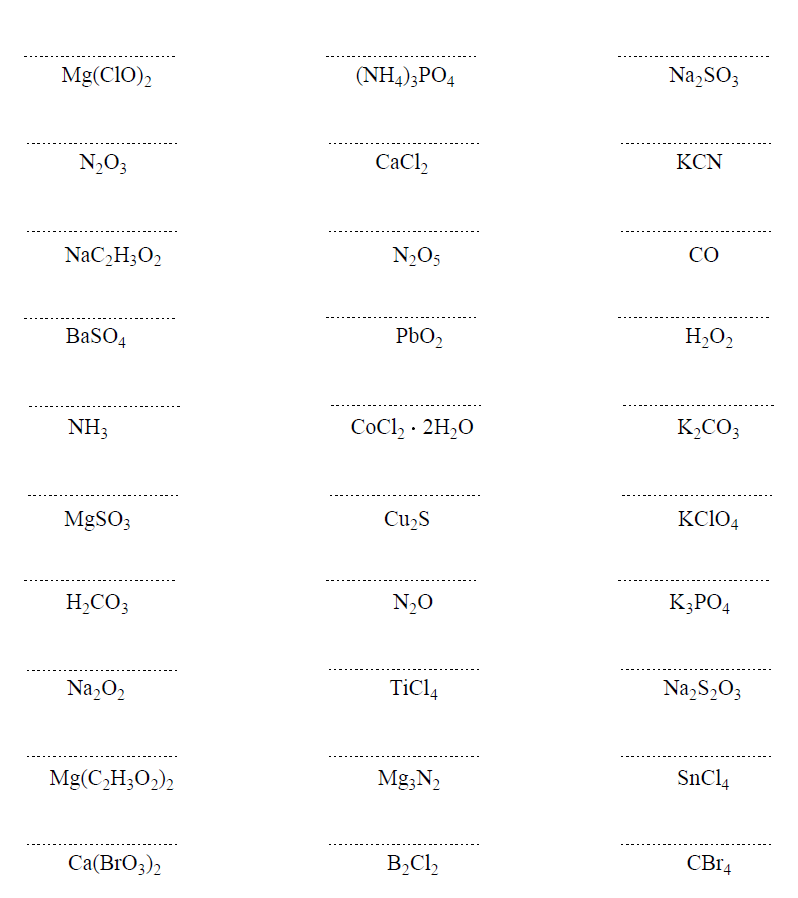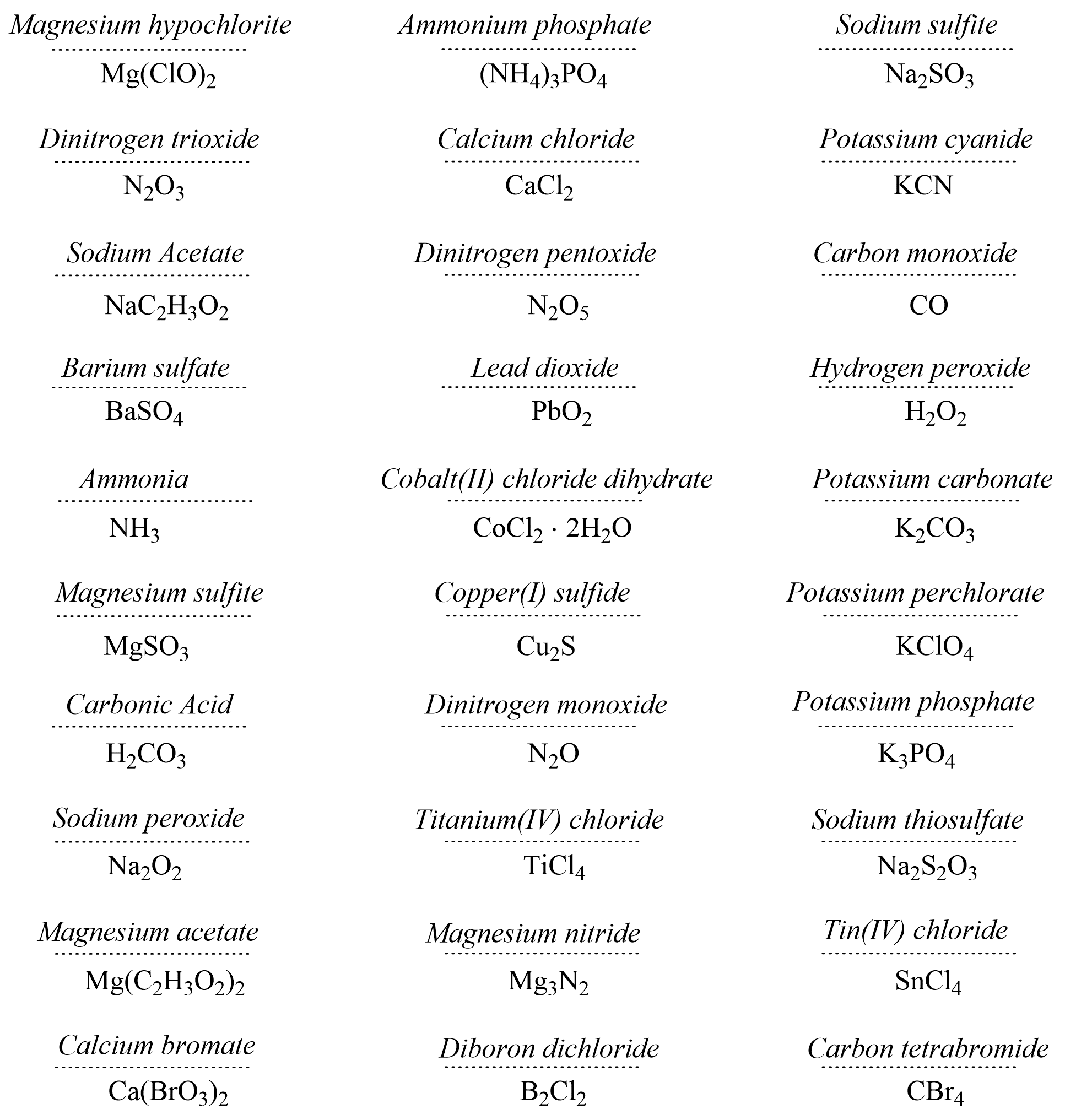3.

Write the formulas of the compounds formed from the following ions: